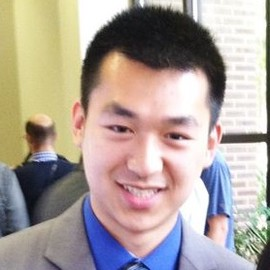# Challenging Common Assumptions in the Unsupervised Learning of Disentangled Representations

Locatello et al., 2019

## Summary

• Raises concerns about the authenticity of recent progress in the unsupervised learning of disentangled representations
• Show theoretically that unsupervised learning is impossible without inductive biases
• Empirical results show that increased disentanglement does not reduce sample complexity of downstream learning
• Disentanglement learning should be explicit about inductive biases, supervision, and concrete benefits of the learned representation
• Links: [ website ] [ pdf ]

## Background

• Core assumption in representation learning: high-dimensional real-world observations are generated from much lower dimensional, semantically meaninful latent variable
• Disentangled representations should therefore separate out these distinct factors of variation in the data
• Additional assumption that disentangled representations will be useful for downstream tasks
• Independent component analysis (ICA) also aims to uncover independent components of the input
• Limited utility for non-linear cases

## Methods

• Considered the following methods, based on VAE loss with regularizer:
• $\beta$-VAE: constrain capacity of bottleneck with hyperparameter in front of KL regularizer
• AnnealedVAE: gradually increases bottleneck capacity
• FactorVAE: penalize total correlation with adversarial training
• $\beta$-TCVAE: penalize correlation with biased MC estimator
• DIP-VAE-II: penalize mismatch between posterior and prior
• Each method uses same architecture, optimizer, and hyperparameters for optimizer and batch size

## Results

• Datasets:
• Deterministic function of latent variable
• dSprites
• Cars3D
• SmallNORB
• Shapes3D
• Stochastic:
• Color-dSprites: random color
• Noisy-dSprites: white shapes on noisy background
• Scream-dSprites: background replaced with random patch with random tint from The Scream painting
• Metrics of disentanglement:
• BetaVAE: accuracy of linear classifier on predicting index of fixed factor of variation
• FactorVAE: majority vote classifier on different feature vector, addresses issues with BetaVAE
• Mutual Information Gap (MIG): normalized gap in MI between highest and second highest coordinate in representation
• Modularity: each dimension of representation depends on at most one factor of variation
• DCI Disentanglement: entropy of distribution from normalizing importance of repsentation dimensions for predicting variation factors
• SAP score: average difference of prediciton error of two most predictive latent dimensions
• Proof that for any marginal distribution of input data, there exists generative models with latent variables disentangled from the learned representation, but aso ones that are completely entangled
• Correct model cannot be determined from just the input distribution
• Results on Color-dSprites show that, in general, the methods produce an aggregated posterior whose individual dimensions are uncorrelated, but not for dimensions of the mean representation
• With the exception of Modularity, all metrics seem to be correlated accross multiple datasets
• Calculate FactorVAE for each method on Cars3D while varying hyperparameters and random seed:
• Large overlap between models suggest hyperparameters and random seed more importaant than specific objective function
• There is significant variation from random seed alone
• Probability of a selected model performing better than a random model on a random dataset and metric is basically at chance
• Plot of sample efficiency vs FactorVAE score does not show a strong correlation

## Conclusion

• Easy to draw incorrect conclusions from results using only a few methods, metrics, and datasets
• Unsupervised model selection remains an open problem
• Poor correlation of sample complexity vs disentanglement might just be due to the tested models’ inability to reliably produce disentangled representations